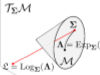### Abstract

In many sensing and control applications, data are represented in the form of symmetric positive definite (SPD) matrices. Considering the underlying geometry of this data space can be beneficial in many robotics applications. In this paper, we present an extension of Gaussian mixture regression (GMR) with input and/or output data on SPD manifolds. As the covariance of SPD datapoints is a 4th-order tensor, we develop a method for parallel transport of high order covariances on SPD manifolds. The proposed approach is experimented in the context of prosthetic hands, with the estimation of wrist movements based on spatial covariance features computed from sEMG signals.

### Bibtex reference

@inproceedings{Jaquier17IROS,
author="Jaquier, N. and Calinon, S.",
title="Gaussian Mixture Regression on Symmetric Positive Definite Matrices Manifolds: Application to Wrist Motion Estimation with {sEMG}",
booktitle="Proc. {IEEE/RSJ} Intl Conf. on Intelligent Robots and Systems ({IROS})",
year="2017",
month="September",
}